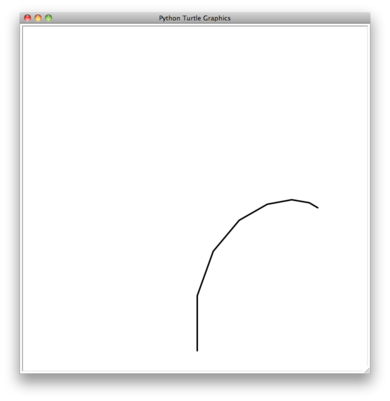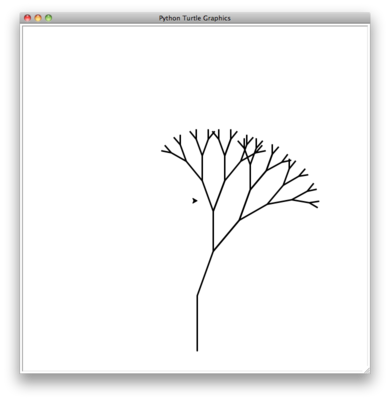# 5.7. Introduction: Visualizing Recursion¶

In the previous section we looked at some problems that were easy to solve using recursion; however, it can still be difficult to find a mental model or a way of visualizing what is happening in a recursive function. This can make recursion difficult for people to grasp. In this section we will look at a couple of examples of using recursion to draw some interesting pictures. As you watch these pictures take shape you will get some new insight into the recursive process that may be helpful in cementing your understanding of recursion.

The tool we will use for our illustrations is Python’s turtle graphics module called turtle. The turtle module is standard with all versions of Python and is very easy to use. The metaphor is quite simple. You can create a turtle and the turtle can move forward, backward, turn left, turn right, etc. The turtle can have its tail up or down. When the turtle’s tail is down and the turtle moves it draws a line as it moves. To increase the artistic value of the turtle you can change the width of the tail as well as the color of the ink the tail is dipped in.

Here is a simple example to illustrate some turtle graphics basics. We will use the turtle module to draw a spiral recursively. ActiveCode 1 shows how it is done. After importing the turtle module we create a turtle. When the turtle is created it also creates a window for itself to draw in. Next we define the drawSpiral function. The base case for this simple function is when the length of the line we want to draw, as given by the len parameter, is reduced to zero or less. If the length of the line is longer than zero we instruct the turtle to go forward by len units and then turn right 90 degrees. The recursive step is when we call drawSpiral again with a reduced length. At the end of ActiveCode 1 you will notice that we call the function myWin.exitonclick(), this is a handy little method of the window that puts the turtle into a wait mode until you click inside the window, after which the program cleans up and exits.

That is really about all the turtle graphics you need to know in order to make some pretty impressive drawings. For our next program we are going to draw a fractal tree. Fractals come from a branch of mathematics, and have much in common with recursion. The definition of a fractal is that when you look at it the fractal has the same basic shape no matter how much you magnify it. Some examples from nature are the coastlines of continents, snowflakes, mountains, and even trees or shrubs. The fractal nature of many of these natural phenomenon makes it possible for programmers to generate very realistic looking scenery for computer generated movies. In our next example we will generate a fractal tree.

To understand how this is going to work it is helpful to think of how we might describe a tree using a fractal vocabulary. Remember that we said above that a fractal is something that looks the same at all different levels of magnification. If we translate this to trees and shrubs we might say that even a small twig has the same shape and characteristics as a whole tree. Using this idea we could say that a tree is a trunk, with a smaller tree going off to the right and another smaller tree going off to the left. If you think of this definition recursively it means that we will apply the recursive definition of a tree to both of the smaller left and right trees.

Let’s translate this idea to some Python code. Listing 1 shows how we can use our turtle to generate a fractal tree. Let’s look at the code a bit more closely. You will see that on lines 5 and 7 we are making a recursive call. On line 5 we make the recursive call right after the turtle turns to the right by 20 degrees; this is the right tree mentioned above. Then in line 7 the turtle makes another recursive call, but this time after turning left by 40 degrees. The reason the turtle must turn left by 40 degrees is that it needs to undo the original 20 degree turn to the right and then do an additional 20 degree turn to the left in order to draw the left tree. Also notice that each time we make a recursive call to tree we subtract some amount from the branchLen parameter; this is to make sure that the recursive trees get smaller and smaller. You should also recognize the initial if statement on line 2 as a check for the base case of branchLen getting too small.

Listing 1

 1 2 3 4 5 6 7 8 9 def tree(branchLen,t): if branchLen > 5: t.forward(branchLen) t.right(20) tree(branchLen-15,t) t.left(40) tree(branchLen-10,t) t.right(20) t.backward(branchLen) 

The complete program for this tree example is shown in ActiveCode 2. Before you run the code think about how you expect to see the tree take shape. Look at the recursive calls and think about how this tree will unfold. Will it be drawn symmetrically with the right and left halves of the tree taking shape simultaneously? Will it be drawn right side first then left side?

Notice how each branch point on the tree corresponds to a recursive call, and notice how the tree is drawn to the right all the way down to its shortest twig. You can see this in Figure 1. Now, notice how the program works its way back up the trunk until the entire right side of the tree is drawn. You can see the right half of the tree in Figure 2. Then the left side of the tree is drawn, but not by going as far out to the left as possible. Rather, once again the entire right side of the left tree is drawn until we finally make our way out to the smallest twig on the left.Figure 1: The Beginning of a Fractal TreeFigure 2: The First Half of the Tree

This simple tree program is just a starting point for you, and you will notice that the tree does not look particularly realistic because nature is just not as symmetric as a computer program. The exercises at the end of the chapter will give you some ideas for how to explore some interesting options to make your tree look more realistic.

Self Check

Modify the recursive tree program using one or all of the following ideas:

• Modify the thickness of the branches so that as the branchLen gets smaller, the line gets thinner.

• Modify the color of the branches so that as the branchLen gets very short it is colored like a leaf.

• Modify the angle used in turning the turtle so that at each branch point the angle is selected at random in some range. For example choose the angle between 15 and 45 degrees. Play around to see what looks good.

• Modify the branchLen recursively so that instead of always subtracting the same amount you subtract a random amount in some range.